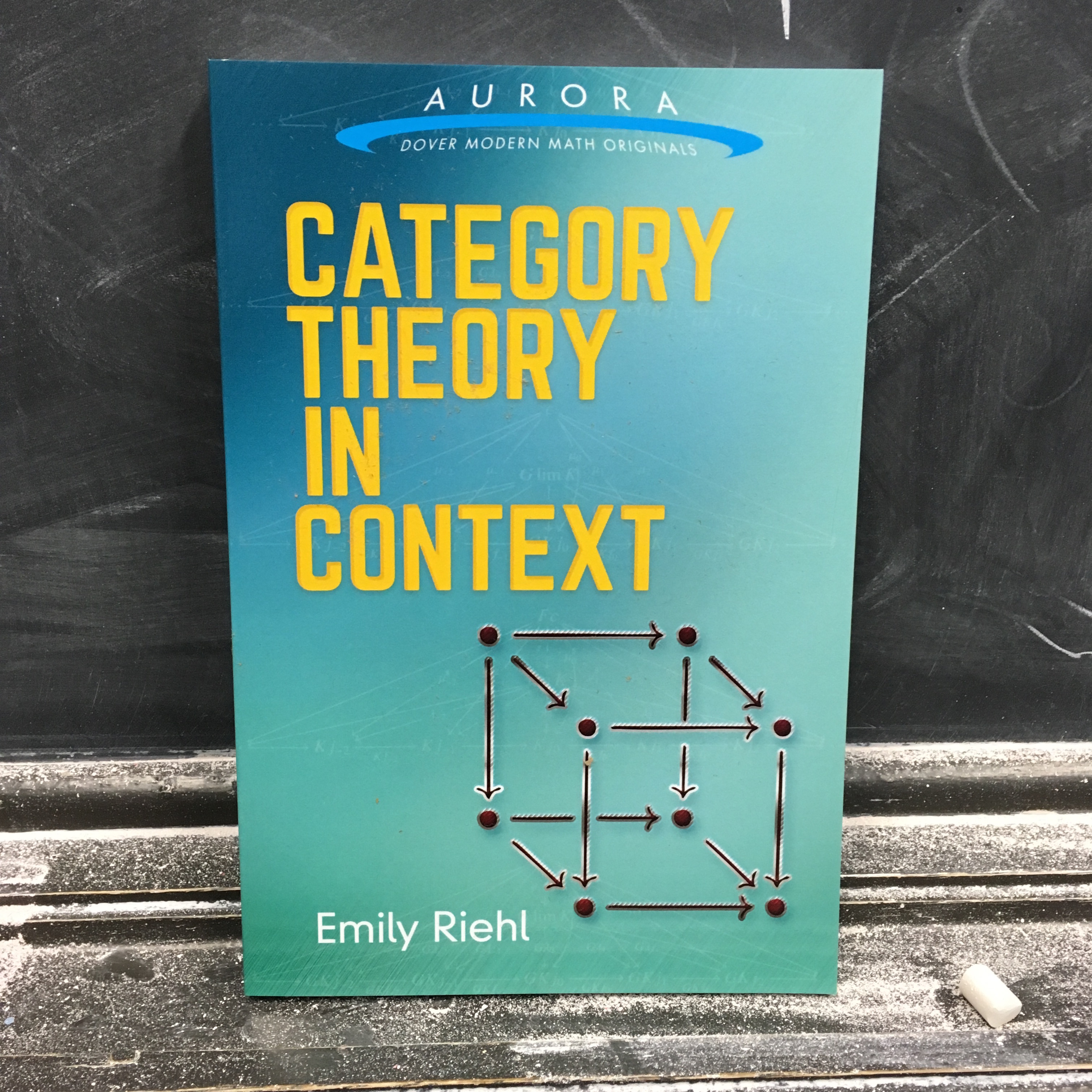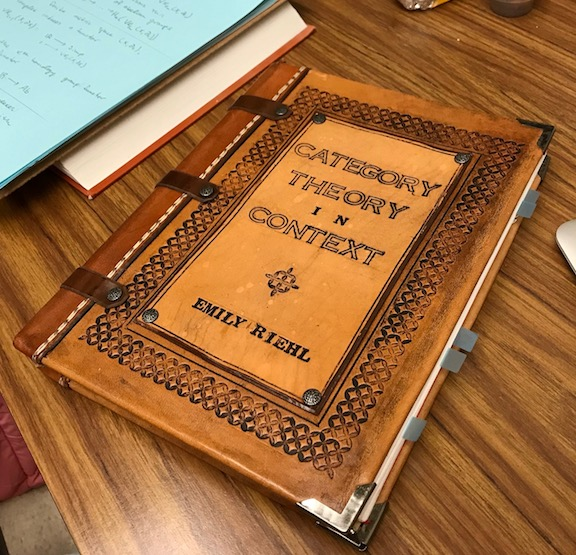## Category Theory in ContextMy second book, Category Theory in Context, originated as lecture notes written for an undergraduate topics course taught at Harvard in the Spring of 2015, and taught against at Johns Hopkins at the graduate level in the Fall of 2015. The book was published in November 2016 by Dover Publications in their new Aurora: Modern Math Originals series.

Thanks to a special arrangement with Dover, I am also able to host a free PDF copy, which can be found here. This version is free to view and download for personal use only. Not for re-distribution, re-sale or use in derivative works. © Emily Riehl 2016. But readers are encouraged to print and bind their own copies (thanks to Riley Zeigler for sharing this photo of her beautiful work):A discussion of the writing process and brief description of the contents can be found on the n-Category Café.

BLURB

Category theory has provided the foundations for many of the twentieth century's greatest advances in pure mathematics. This concise, original text for a one-semester introduction to the subject is derived from courses that author Emily Riehl taught at Harvard and Johns Hopkins Universities. The treatment introduces the essential concepts of category theory: categories, functors, natural transformations, the Yoneda lemma, limits and colimits, adjunctions, monads, Kan extensions, and other topics.

Suitable for advanced undergraduates and graduate students in mathematics, the text provides tools for understanding and attacking difficult problems in algebra, number theory, algebraic geometry, and algebraic topology. Drawing upon a broad range of mathematical examples from the categorical perspective, the author illustrates how the concepts and constructions of category theory arise from and illuminate more basic mathematical ideas. While the reader will be rewarded for familiarity with these background mathematical contexts, essential prerequisites are limited to basic set theory and logic.

REVIEWS

Karo's review frames the issue quite nicely:

The Mathemagician’s Axiom of Textbook Prerequisites: Let M be the minimum actual prerequisites for an average student being able to read and understand a given presentation of a subject in a mathematics textbook. Let A be the author’s stated prerequisites. Then usually: A <<<<<<< M

Every expository project is written with a particular reader in mind, and I realize now that in the published blurb it would have been more useful to describe the target audience for the book, rather than the essential prerequisites. My intended audience might be described as peri-graduate students, ranging from advanced mathematics undergraduates, to graduate students, to perhaps even research mathematicians in other disciplines. In particular, the readers I had in mind were self-identified mathematicians — not to suggest by any means that these are the only people interested in learning category theory. However, the goal of this particular expository project was somewhat narrower: to motivate categorical concepts for those who are already high on abstract mathematics and might be tempted to take in the view from one level further up in the stratosphere.

When I taught category theory to undergraduates, I asked that students had taken a semester-long undergraduate course in point-set topology and a year-long undergraduate course in abstract algebra, perhaps running concurrently. Neither of these topics are “essential prerequisites” to learning category theory, but prior acquaintance with algebra and topology is invaluable for appreciating what category theory is useful for in mathematics.

As with any specialized mathematics textbook, the real prerequisite qualification is some level of “mathematical sophistication” — meaning comfort with abstract definitions, mathematical reasoning and common turns of phrase, and crucially also the confidence to push through discussions that don’t make any sense. In class, I told my students to treat all of the examples this way, as offerings at a banquet to sample from at will and also skip over without anxiety. My hope was that particular digressions that I wanted to include for color could be bracketed and left aside without causing too much intimidation.

Perhaps the strongest illustration of this is the rather unfortunately positioned first page of the first chapter, which several reviewers have complained about. There, I'm trying to describe the historical origins of the subject, which arose from a research question contemplated by the founders Eilenberg and Mac Lane that is inevitably rather more complicated than the examples that are the focus of the rest of the book. While I suspect that many of the technicalities in my description of that problem are somewhat opaque, I hope that what I've written nonetheless is clear enough in outline to set the scene. To get a sense of the level at which the book is written, sections 1.1-1.3 are more indicative of what will follow in the rest of the text.

CONTENTS

The preface begins by whetting the readers appetite with a list of sample corollaries of categorical theorems, for instance in a path-connected space, any choice of basepoint yields an isomorphic fundamental group. Also, every row operation on matrices with n rows is defined by left multiplication by some n × n matrix, namely the matrix obtained by performing the row operation on the identity matrix.

The first chapter introduces categories, functors, and natural transformations. The advantages of knowing the functoriality of a given mathematical construction are illustrated by proving the Brouwer Fixed Point Theorem: that any continuous endomorphism of a disk must have a fixed point. Before proving the characterization of those functors that define an equivalence of categories, some attempt is made to describe the basic techniques involved in the “art of the diagram chase.”

The second chapter discusses universal properties, representability, and the Yoneda lemma. The motivating example is the (contravariant) functor that sends a graph to its set of vertex colorings with n colors. This functor is represented by the complete graph Kn on n elements, graph homomorphisms GKn defining n-colorings of the vertices of G. The final section of this chapter proves that universal objects can be understood as objects that are either initial or terminal in an appropriate category, namely the category of elements of the functor that they represent.

The third chapter introduces limits and colimits as a special case of objects defined by a universal property. For sake of expositional diversity, I deduce the usual construction of a generic colimit as a coequalizer of a pair of maps between two coproducts as a corollary of the Yoneda lemma and the corresponding fact for limits of diagrams valued in Set (which can be proven by comparing the elements of the two limit constructions).

The fourth chapter studies adjoint functors in their many guises. Several of the motivating corollaries in the preface reappear as a special case of the fact that right adjoints preserve limits (the proof of which is displayed as a watermark on the cover of the book). A final section on the adjoint functor theorems explains how a special case of the general adjoint functor theorem can be used to construct the free group on a set and that the special adjoint functor theorem can be understood as an abstraction of the construction of the Stone-Čech compactification.

The fifth chapter presents categorical universal algebra in the guise of monads, listing several naturally-arising examples of categories of algebras and Kleisli categories: for instance, the Kleisli category for the Giry monad defines the category of measurable spaces and Markov kernels. After proving the monadicity theorem, a final section gives an explicit construction of limits and colimits in a category of algebras, with the construction of the free product of groups used to motivate the general coproduct formula.

The final chapter, whose irresistible title is borrowed from Mac Lane’s Categories for the Working Mathematician, studies Kan extensions both in category theory and in the wild (e.g., in the guise of induced representations) and introduces derived functors. This chapter is a reworking of the first chapter of my previous book, Categorical Homotopy Theory, with several expository improvements.

A cheeky epilogue lists theorems in category theory, as a rejoinder to those who still claim that there are none.

SUPPLEMENTAL RESOURCES